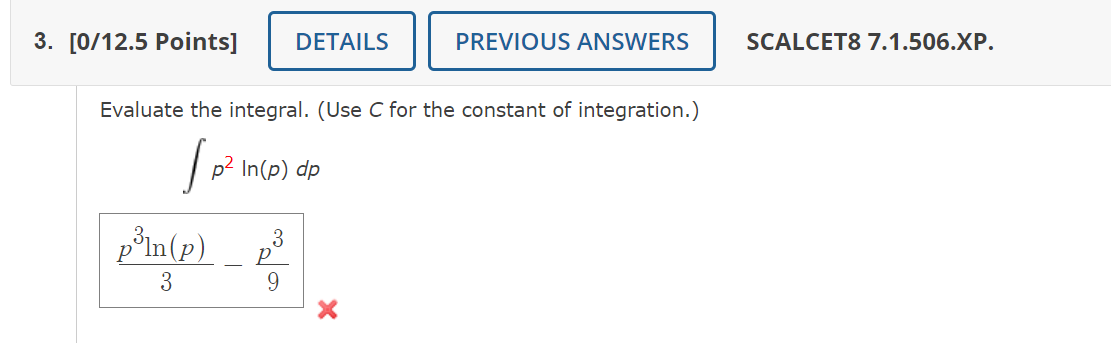Home / Expert Answers / Calculus / evaluate-the-integral-use-c-for-the-constant-of-integration-int-p-2-ln-p-d-p-pa560

# (Solved): Evaluate the integral. (Use $$C$$ for the constant of integration.) $\int p^{2} \ln (p) d p$ ...Evaluate the integral. (Use $$C$$ for the constant of integration.) $\int p^{2} \ln (p) d p$ $\frac{p^{3} \ln (p)}{3}-\frac{p^{3}}{9}$

We have an Answer from Expert